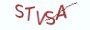Java基础教程

# 5.5.1 理解数据运算1+1之所以等于2，是因为我们先有了物物相加的生活经验，然后以数字符号的形式来表示这种算数逻辑的处理过程。

# 5.5.3 Java中的数据运算

Java中的数据运算主要有赋值运算，算数运算，关系运算，逻辑运算。

(1) 赋值运算

```// 定义布尔类型变量is_happy,初始化为true
boolean is_happy = true;
// 将变量is_happy赋值为false
is_happy = false;```

(2) 算数运算

 算数运算符 描述 + 加法运算 - 减法运算 * 乘法运算 / 除法运算 % 取余运算 ++ 自增运算 -- 自减运算

```/**
* @author 薯条老师
* @date  2021-08-17
* @version 1.0
*/
public class HelloJava {
public  static void main(String[] args) {
// 定义year变量，值为2020
int year = 2020;
// 定义sum变量，用来保存数字各位的和
int sum = 0;
// 1. 除以1000，得到千位数
int number = year/1000;
// 与千位数相加
sum = sum+number;
// 2. 与1000取余，去掉千位数
year = year % 1000;
// 除以100，得到百位数
number = year / 100;
// 与百位数相加
sum = sum+number;
// 3. 与100取余，去掉百位数
year = year % 100;
// 除以10，得到十位数
number = year / 10;
// 与十位数相加
sum = sum+number;
// 4. 与10取余，去掉个位数
year = year % 10;
// 除以1，得到个位数
number = year / 1;
// 与个位数相加
sum = sum+number;
System.out.println(sum);
}
}```

（1）将数字与各数位的基数作整除运算，可以获取当前数位的数字，例如千位数的基数为1000，百位数的基数为100，十位数的基数为10，以此类推。将2020与1000进行整除，结果为2，显然2就是千位数的数字。

（2）将数字与数位的基数进行求余运算，可获得下一数位的数。例如2121与1000进行求余运算，余数为121。如果余数小于数位的基数，那么说明该数位的数字为0。例如2020与1000进行求余运算，余数为20，20小于100，那么百位上的数字为0。

```public class HelloJava {
public  static void main(String[] args) {
// 定义整型变量number,初始化为0
int number = 0;
// 使用后缀自增运算，先赋值后自增
int value = number++;
System.out.println("value的值:"+value+" number的值:"+number);
}
}```

value的值:0 number的值:1

```public class HelloJava {
public  static void main(String[] args) {
// 定义整型变量number,初始化为1
int number = 1;
// 使用前缀自减运算，先自减后赋值
int value = --number;
System.out.println("value的值:"+value+" number的值:"+number);
}
}```

value的值:0 number的值:0

```public class HelloJava {
public  static void main(String[] args) {
int number = 0;
// number+=2 等价于number=number+2
number +=2;
System.out.println("number的值:"+number);
}
}```

(1) 定义了一个number变量，值为0

(2) number += 2等价于number = number + 2，先将numbe的值与2进行加法运算，再将

(3)其它的算数运算同样可以与赋值运算组成复合运算，以此类推。

(3) 关系运算

 关系运算符 描述 > 比较左操作数是否大于右操作数 < 比较左操作数是否小于右操作数 == 比较左操作数是否与右操作数相等 >= 比较左操作数是否大于等于右操作数 <= 比较左操作数是否小于等于右操作数 != 比较左操作数是否不等于右操作数

```public class HelloJava {
public  static void main(String[] args) {
int x = 1;
int y = 2;
// 判断x是否大于y,输出的结果为布尔类型
boolean result = x > y;
// result的值为false
System.out.println("result的值:"+ result);
}
}```

(4) 逻辑运算

 逻辑运算符 描述 && 逻辑与运算，所有表达式的值为真值时，结果才为真。 || 逻辑或运算，只要其中一个为真，那么结果就为真。 ！ 逻辑非运算，非真就是假，非假就是真。

# 5.5.4 结合性与优先级

```int x =3;
int y = 5;
int z = 7;
// 赋值运算为右结合性，按从右到左的顺序来赋值
x = y = z = 10;
// 经过以上赋值以后,x,y,z的值均为10```

(1) 赋值运算的结合性为从右至左，所以首先赋值的是z=10。

(2) 然后将变量z的值再赋值给变量y,将变量y的值再赋值给x

```public class HelloJava {
public  static void main(String[] args) {
int x =3;
int y = 5;
int z = 7;
// 优先计算y*z，再与x相加
int sum = x+y*z;
System.out.println("sum的值:"+sum);
}
}```

(1) 乘法的优先级最高，优先计算y*z，结果为35，然后再计算x+35的值

(2) x的值为3，所以最终的值为38

```public class HelloJava {
public  static void main(String[] args) {
int x =3;
int y = 5;
int z = 7;
// 优先计算x+y，再与z相乘
int sum = (x+y)*z;
System.out.println("sum的值:"+sum);
}
}```

(1) 先计算()里面的x+y的值,因为()里面的优先级最高

(2) 再将x+y的值与z相乘，最终结果为56

# 5.5.5 课后习题

(1) Java中主要有哪些数据运算？

(2) 简述你对短路逻辑的理解，并举出一个生活中的例子。

(3) 如果赋值运算为左结合性，那么在5.5.4节的代码实例中x,y,z的值分别为多少？

(4) 编写一段简单的代码，以测试赋值运算的优先级

# 5.5.6 高薪就业班

`(1) Python后端工程师高薪就业班，月薪10K-15K，免费领取课程大纲(2) Python爬虫工程师高薪就业班，年薪十五万，免费领取课程大纲(3) Java后端开发工程师高薪就业班，月薪10K-20K, 免费领取课程大纲(4) Python大数据工程师就业班，月薪12K-25K,免费领取课程大纲`## 注册账号